# Mandelbrot Set of Quadratic Rational Functions

## in the Fractal Gallery of William Gilbert

Pure Mathematics Department, University of Waterloo, Ontario, Canada

## Mathematical Description

The general quadratic rational function can be written in the form
F ( z ) = ( 1 / a ) ( z + b + ( 1 / z ) )
with two complex parameters a and b.

The Mandelbrot set consists of the parameters ( a, b ), in 4-dimensional space, for which the filled-in Julia set is connected. We illustrate various 2-dimensional sections of this 4-dimensional set where

b = r a + s       with r and s constants.

The function F( z ) has critical points at 1 and -1, and one of its three fixed points at infinity. Infinity is an attractive fixed point if and only if |a| < 1.

## Pictures

The pictures show various plane sections of the Mandelbrot Set of Quadratic Rational Functions.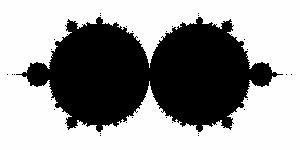The standard Mandelbrot set
This is the section where
b = - a + 2

This is the view shown on Plate 189 of Mandelbrot's book, since the function
F ( z ) = ( 1 / a ) ( z + b + ( 1 / z ))
is conjugate to the function
b z ( 1 - z ) when a + b = 2.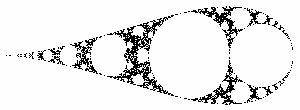Snowshoe
This is the section where
b = - a - 2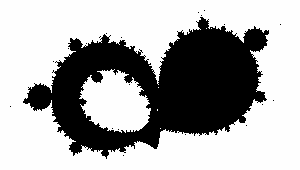Spyglass
This is the a-plane section where
b = ( - 1 + 0.4 i ) a + 2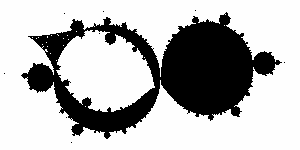Wink
This is the a-plane section where
b = - a + 1.7 + 0.3 i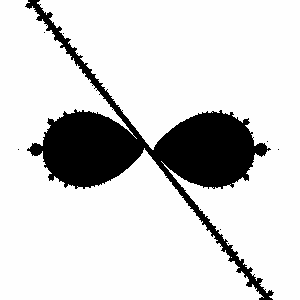Tie and Cane
This is the b-plane section where
a = - b + 50 + 200 i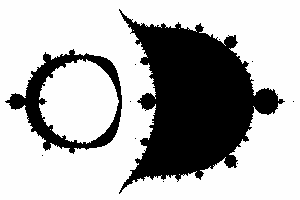Napoleon
This is the a-plane section where
b = - a + 3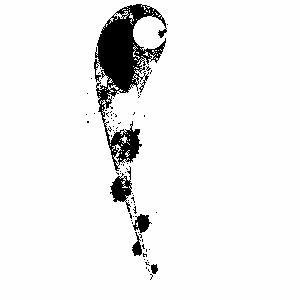Sperm
This is the a-plane section where
b = ( 0.3 - 0.4 i ) a + 1.2 + 0.3 i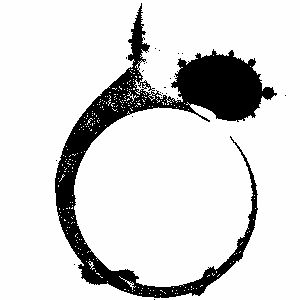Ring
This is the b-plane section where
a = ( 0.3 - 0.4 i ) b + 1.2 + 0.3 i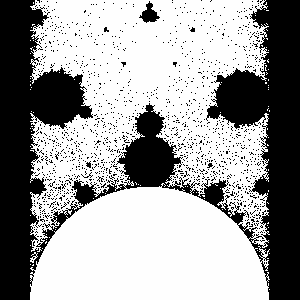Blood Clot
This is the a-plane section where
b = 0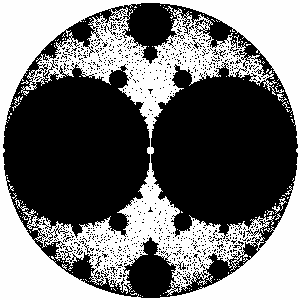Alien
This is the (1/a)-plane section where
b = 0
This is the same set as the previous image, but with a different form of the parameter.Scallops
This is the (1/a)-plane section where
b = 2

## Program

• W.J. Gilbert: Rational Mandelbrot Set Program (RatMandel) written in Objective C for the NeXT computer only and distributed on the Big Green CD ROM 4.0, Skylee Press, San Francisco (1994) [The program with source was on the PEAK and PEANUTS NeXT Archives, but these are gone now.]

## References

• Milnor, John: Geometry and dynamics of quadratic rational maps. Experiment. Math. 2 (1993), no. 1, 37--83.

© 1997 by William Gilbert          Back to the entrance of the Fractal Gallery of William Gilbert.